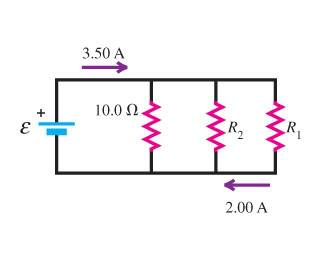# Electrical Circuit and Current

• Soaring Crane

## Homework Statement

In the circuit shown in the figure, the rate at which R1 is dissipating electrical energy is 20.0 W.I just have questions about the circuit's setup. The resistors are arranged in a parallel circuit?

As for the purple arrows, is 2.00 A I_1 and 3.50 A the total current in the circuit?

See below.

## The Attempt at a Solution

This is just a preliminary question. I shall post my workings at a later time if I am baffled by the questions associated with the figure.

Thanks.

Sounds good to me so far.

I just have questions about the circuit's setup. The resistors are arranged in a parallel circuit?
Yes, the resistors are in parallel.

As for the purple arrows, is 2.00 A I_1 and 3.50 A the total current in the circuit?
That's how I would interpret them.